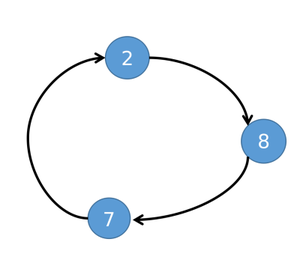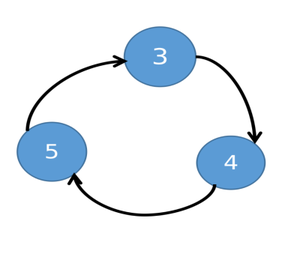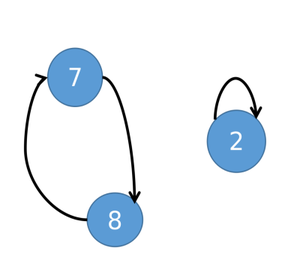# Minimize cost to sort an Array by swapping any pair of element (X, Y) with cost as (X + Y)

• Difficulty Level : Expert
• Last Updated : 20 Aug, 2021

Given an array arr[] consisting of N integers, the task is to find the minimum cost to sort the given array arr[] in ascending order by swapping any pair of elements (X, Y) such that the cost of swapping is (X + Y).

Examples:

Input: arr[] = {3, 2, 1}
Output: 4
Explanation:
Following are the swapping of array elements performed to sort the array:

1. Swapping the array elements at index 0 and 2 modifies the array to {1, 2, 3}. The cost of this swapping operation is (arr + arr) = (3 + 1) = 4.

After the above steps, the given array is sorted and the total cost is 4, which is the minimum among all possible combinations of swapping.

Input: arr[] = {7, 9, 15}
Output: 0

Approach:  The given problem can be solved based on the following observations:

1. Form a directed graph by forming edges between every ith element of the current array and the sorted array.
2. It can be observed that every component will always form a cycle as there every node will have in-degree and out-degree equal to 1.
3. Therefore, the idea is to sort the elements of each cycle separately.

Illustration:

• Suppose the given array is {8, 4, 5, 3, 2, 7}. The sorted array will be equal to the {2, 3, 4, 5, 7, 8}.
• For the above array, the graph will contain two components which are cycles.• If two elements are swapped in a cycle, of length K > 1 such that at least 1 element of this cycle will go to its destination. Then after the swap, it will be split into two cycles, one with length K – 1 and another one with length 1.
• For the given array, If 2 and 8 are swapped of 2 → 8 → 7 → 2 cycles. 2 will go to its destination, and 7 → 8 → 7 will form a smaller cycle.• Therefore, the minimum number of swaps needed to sort a cycle of size K is equal to the (K-1).
• It can be observed that each swap will add two elements to the cost. So 2 × (K – 1) elements will be added to the cost and there are K elements. So some elements will be added multiple times to the cost.
• Therefore, the idea is to, swap with the minimum value of a cycle with every other element to place them at the correct positions. Then in the final cost, every element will be added once and the minimum element will be added K – 1 time. So this is the optimal approach to solve a cycle.
• For sorting a cycle there are two choices: either to use only the local minimum of the cycle or to use both local and overall minimum of the array.

Follow the steps below to solve the problem:

• Initialize a variable res as 0 to store the total cost.
• Copy every element of arr[] to another array, say copyArr[] and sort copyArr[] in ascending order.
• Initialize a hashmap say place and store array elements and the correct position of it in the sorted array as key-value pair.
• Also, Initialize an array, visited[] of size N, and mark all its entries as false.
• Iterate over the array, arr[] using the variable i, and perform the following steps:
• If visited[i] is true then continue.
• If the element visited index is visited true and perform the following steps:
• If place[arr[i]] is equal to i then mark visited[i], true and continue.
• Initialize a variable say min_value as arr[i] and sum as 0, to store the minimum value of the current cycle and the sum of the elements of the cycle.
• Also, initialize a variable j as i to iterate over the cycle and a variable num as 0 to store the count of numbers in the cycle.
• Iterate until visited[j] is not true and in each iteration perform the following steps:
• Increment sum by arr[j] and num by 1 and then mark the visited[j] as true.
• Update min_value to min(min_value, arr[j]). And then assign value of place[arr[j]] to j.
• Decrement sum by the min_value.
• Now find the cost obtained by using the local minimum, min_value, and store it in a variable say Cost1.
• Cost1 = sum + min_val*(num-1).
• Now find the cost obtained by using the global minimum, copyArr, and store it in a variable say Cost2.
• Cost2 = copyArr * (num + 1) + 2 * min_val+ sum
• Now increment res by min(Cost1, Cost2).
• After completing the above steps, print the res as the total cost.

Below is the implementation of the above approach:

## C++

 `// C++ program for the above approach` `#include ``using` `namespace` `std;` `// Function to find the minimum cost to``// sort the array``int` `findMinimumCost(``int` `arr[], ``int` `N)``{``    ``// Stores the required result``    ``int` `res = 0;` `    ``// Create 2 arrays``    ``int` `copyArr[N], visited[N];` `    ``for` `(``int` `i = 0; i < N; ++i) {``        ``copyArr[i] = arr[i];``        ``visited[i] = ``false``;``    ``}` `    ``// Sort the array, copyArr[] in``    ``// increasing order``    ``sort(copyArr, copyArr + N);` `    ``// Map the numbers to their desired``    ``// place after sorting``    ``map<``int``, ``int``> place;` `    ``// Store the original places of the``    ``// elements in map``    ``for` `(``int` `i = 0; i < N; ++i) {``        ``place[copyArr[i]] = i;``    ``}` `    ``// Iterate in the range [0, N-1]``    ``for` `(``int` `i = 0; i < N; ++i) {` `        ``// If the ith index is not visited``        ``if` `(visited[i] == ``false``) {` `            ``// If the original place and``            ``// the place in sorted array``            ``// is same then only mark this``            ``// element as visited``            ``if` `(place[arr[i]] == i) {``                ``visited[i] = ``true``;``                ``continue``;``            ``}` `            ``// Else a new cycle is present``            ``int` `min_val = arr[i], cost1, cost2;``            ``int` `num = 0;``            ``int` `sum = 0;``            ``int` `j = i;` `            ``// Iterate while the nodes``            ``// in the current cycle is``            ``// not visited``            ``while` `(visited[j] == ``false``) {` `                ``// Increment sum by arr[j]``                ``sum += arr[j];``                ``num++;` `                ``// Update the min_val value``                ``if` `(arr[j] < min_val) {``                    ``min_val = arr[j];``                ``}` `                ``// Mark j as visited``                ``visited[j] = ``true``;` `                ``// Place j at its``                ``// original place``                ``j = place[arr[j]];``            ``}` `            ``// Sum of all numbers of``            ``// cycle other than minimum``            ``sum -= min_val;` `            ``// Cost from local minimum``            ``cost1 = sum + min_val * (num - 1);` `            ``// Cost from overall minimum``            ``cost2 = copyArr * (num + 1) + 2 * min_val``                    ``+ sum;` `            ``// Add the lower cost to``            ``// the final result``            ``if` `(cost1 < cost2) {``                ``res += cost1;``            ``}``            ``else` `{``                ``res += cost2;``            ``}``        ``}``    ``}` `    ``// Print the minimum cost``    ``return` `res;``}` `// Driver Code``int` `main()``{``    ``int` `arr[] = { 3, 2, 1 };``    ``int` `N = (``sizeof``(arr) / ``sizeof``(``int``));``    ``cout << findMinimumCost(arr, N);` `    ``return` `0;``}`

## Java

 `import` `java.util.ArrayList;``import` `java.util.Arrays;``import` `java.util.HashMap;``import` `java.util.Map;` `//Java program for above approach``class` `GFG{` `    ``// Function to find the minimum cost to``// sort the array``    ``static` `int` `findMinimumCost(``int``[] arr, ``int` `N)``    ``{``        ``// Stores the required result``        ``int` `res = ``0``;` `        ``// Create 2 arrays``        ``int``[] copyArr = ``new` `int``[N];``        ``boolean``[] visited = ``new` `boolean``[N];` `        ``for` `(``int` `i = ``0``; i < N; ++i) {``            ``copyArr[i] = arr[i];``            ``visited[i] = ``false``;``        ``}` `        ``// Sort the array, copyArr[] in``        ``// increasing order``        ``Arrays.sort(copyArr);` `        ``// Map the numbers to their desired``        ``// place after sorting``        ``Map place = ``new` `HashMap<>();` `        ``// Store the original places of the``        ``// elements in map``        ``for` `(``int` `i = ``0``; i < N; ++i) {``            ``place.put(copyArr[i],i);``        ``}` `        ``// Iterate in the range [0, N-1]``        ``for` `(``int` `i = ``0``; i < N; ++i) {` `            ``// If the ith index is not visited``            ``if` `(visited[i] == ``false``) {` `                ``// If the original place and``                ``// the place in sorted array``                ``// is same then only mark this``                ``// element as visited``                ``if` `(place.get(arr[i]) == i) {``                    ``visited[i] = ``true``;``                    ``continue``;``                ``}` `                ``// Else a new cycle is present``                ``int` `min_val = arr[i], cost1, cost2;``                ``int` `num = ``0``;``                ``int` `sum = ``0``;``                ``int` `j = i;` `                ``// Iterate while the nodes``                ``// in the current cycle is``                ``// not visited``                ``while` `(visited[j] == ``false``) {` `                    ``// Increment sum by arr[j]``                    ``sum += arr[j];``                    ``num++;` `                    ``// Update the min_val value``                    ``if` `(arr[j] < min_val) {``                        ``min_val = arr[j];``                    ``}` `                    ``// Mark j as visited``                    ``visited[j] = ``true``;` `                    ``// Place j at its``                    ``// original place``                    ``j = place.get(arr[j]);``                ``}` `                ``// Sum of all numbers of``                ``// cycle other than minimum``                ``sum -= min_val;` `                ``// Cost from local minimum``                ``cost1 = sum + min_val * (num - ``1``);` `                ``// Cost from overall minimum``                ``cost2 = copyArr[``0``] * (num + ``1``) + ``2` `* min_val``                        ``+ sum;` `                ``// Add the lower cost to``                ``// the final result``                ``if` `(cost1 < cost2) {``                    ``res += cost1;``                ``}``                ``else` `{``                    ``res += cost2;``                ``}``            ``}``        ``}` `        ``// Print the minimum cost``        ``return` `res;``    ``}` `    ``// Driver Code``    ``public` `static` `void` `main(String[] args) {``        ``int``[] arr = { ``3``, ``2``, ``1` `};``        ``int` `N = arr.length;``        ``System.out.println(findMinimumCost(arr, N));` `    ``}``}` `// This code is contributed by hritikrommie.`

## Python3

 `# Python program for the above approach` `# Function to find the minimum cost to``# sort the array``def` `findMinimumCost(arr, N):``    ``# Stores the required result``    ``res ``=` `0` `    ``# Create 2 arrays``    ``copyArr ``=` `[``0``] ``*` `N``    ``visited ``=` `[``0``] ``*` `N` `    ``for` `i ``in` `range``(N):``        ``copyArr[i] ``=` `arr[i]``        ``visited[i] ``=` `False` `    ``# Sort the array, copyArr[] in``    ``# increasing order``    ``copyArr.sort()` `    ``# Map the numbers to their desired``    ``# place after sorting``    ``place ``=` `{}` `    ``# Store the original places of the``    ``# elements in map``    ``for` `i ``in` `range``(N):``        ``place[copyArr[i]] ``=` `i` `    ``# Iterate in the range [0, N-1]``    ``for` `i ``in` `range``(N):` `        ``# If the ith index is not visited``        ``if` `(visited[i] ``=``=` `False``):` `            ``# If the original place and``            ``# the place in sorted array``            ``# is same then only mark this``            ``# element as visited``            ``if` `(place[arr[i]] ``=``=` `i):``                ``visited[i] ``=` `True``                ``continue` `            ``# Else a new cycle is present``            ``min_val ``=` `arr[i]``            ``num ``=` `0``            ``sum` `=` `0``            ``j ``=` `i` `            ``# Iterate while the nodes``            ``# in the current cycle is``            ``# not visited``            ``while` `(visited[j] ``=``=` `False``):` `                ``# Increment sum by arr[j]``                ``sum` `+``=` `arr[j]``                ``num ``+``=` `1` `                ``# Update the min_val value``                ``if` `(arr[j] < min_val):``                    ``min_val ``=` `arr[j]` `                ``# Mark j as visited``                ``visited[j] ``=` `True` `                ``# Place j at its``                ``# original place``                ``j ``=` `place[arr[j]]` `            ``# Sum of all numbers of``            ``# cycle other than minimum``            ``sum` `-``=` `min_val` `            ``# Cost from local minimum``            ``cost1 ``=` `sum` `+` `min_val ``*` `(num ``-` `1``)` `            ``# Cost from overall minimum``            ``cost2 ``=` `copyArr[``0``] ``*` `(num ``+` `1``) ``+` `2` `*` `min_val ``+` `sum` `            ``# Add the lower cost to``            ``# the final result``            ``if` `(cost1 < cost2):``                ``res ``+``=` `cost1``            ``else``:``                ``res ``+``=` `cost2` `    ``# Print the minimum cost``    ``return` `res`  `# Driver Code` `arr ``=` `[``3``, ``2``, ``1``]``N ``=` `len``(arr)``print``(findMinimumCost(arr, N))`  `# This code is contributed by gfgking`

## C#

 `// C# program for the above approach``using` `System;``using` `System.Collections.Generic;` `class` `GFG{``    ` `// Function to find the minimum cost to``// sort the array``static` `int` `findMinimumCost(``int``[] arr, ``int` `N)``{``    ` `    ``// Stores the required result``    ``int` `res = 0;` `    ``// Create 2 arrays``    ``int``[] copyArr = ``new` `int``[N];``    ``int``[] visited = ``new` `int``[N];` `    ``for``(``int` `i = 0; i < N; ++i)``    ``{``        ``copyArr[i] = arr[i];``        ``visited[i] = 0;``    ``}` `    ``// Sort the array, copyArr[] in``    ``// increasing order``    ``Array.Sort(copyArr);` `    ``// Map the numbers to their desired``    ``// place after sorting``    ``Dictionary<``int``,``               ``int``> place = ``new` `Dictionary<``int``,``                                           ``int``>();` `    ``// Store the original places of the``    ``// elements in map``    ``for``(``int` `i = 0; i < N; ++i)``    ``{``        ``place[copyArr[i]] = i;``    ``}` `    ``// Iterate in the range [0, N-1]``    ``for``(``int` `i = 0; i < N; ++i)``    ``{``        ` `        ``// If the ith index is not visited``        ``if` `(visited[i] == 0)``        ``{``            ` `            ``// If the original place and``            ``// the place in sorted array``            ``// is same then only mark this``            ``// element as visited``            ``if` `(place[arr[i]] == i)``            ``{``                ``visited[i] = 1;``                ``continue``;``            ``}` `            ``// Else a new cycle is present``            ``int` `min_val = arr[i], cost1, cost2;``            ``int` `num = 0;``            ``int` `sum = 0;``            ``int` `j = i;` `            ``// Iterate while the nodes``            ``// in the current cycle is``            ``// not visited``            ``while` `(visited[j] == 0)``            ``{``                ` `                ``// Increment sum by arr[j]``                ``sum += arr[j];``                ``num++;` `                ``// Update the min_val value``                ``if` `(arr[j] < min_val)``                ``{``                    ``min_val = arr[j];``                ``}` `                ``// Mark j as visited``                ``visited[j] = 1;` `                ``// Place j at its``                ``// original place``                ``j = place[arr[j]];``            ``}` `            ``// Sum of all numbers of``            ``// cycle other than minimum``            ``sum -= min_val;` `            ``// Cost from local minimum``            ``cost1 = sum + min_val * (num - 1);` `            ``// Cost from overall minimum``            ``cost2 = copyArr * (num + 1) +``                             ``2 * min_val + sum;` `            ``// Add the lower cost to``            ``// the final result``            ``if` `(cost1 < cost2)``            ``{``                ``res += cost1;``            ``}``            ``else``            ``{``                ``res += cost2;``            ``}``        ``}``    ``}` `    ``// Print the minimum cost``    ``return` `res;``}``    ` `// Driver code``public` `static` `void` `Main()``{``    ``int``[] arr = { 3, 2, 1 };``    ``int` `N = arr.Length;``    ` `    ``Console.WriteLine(findMinimumCost(arr, N));``}``}` `// This code is contributed by sanjoy_62`

## Javascript

 ``

Output:

`4`

Time Complexity: O(N*log N)
Auxiliary Space: O(N)

My Personal Notes arrow_drop_up